Home

Separate Fraction Bars Items for Grades 3 and 4

Each of the following items is described below and is available separately.

(1) Grades 3-4 Fraction Bars Teacher’s Guide(lessons, games, and masters)
(2) Deck of Fraction Bars
(49 bars)
(3) Deck of Fraction Bars Playing Cards
(49 cards)
(4) Grades 3-4 Fraction Bars Teacher Resource Package
(5) Fraction Bars Transparencies
(49 transparent bars)
(6) Fraction Bars Activity Mats
(30 mats)
(7) Game Markers
(200 markers in four colors)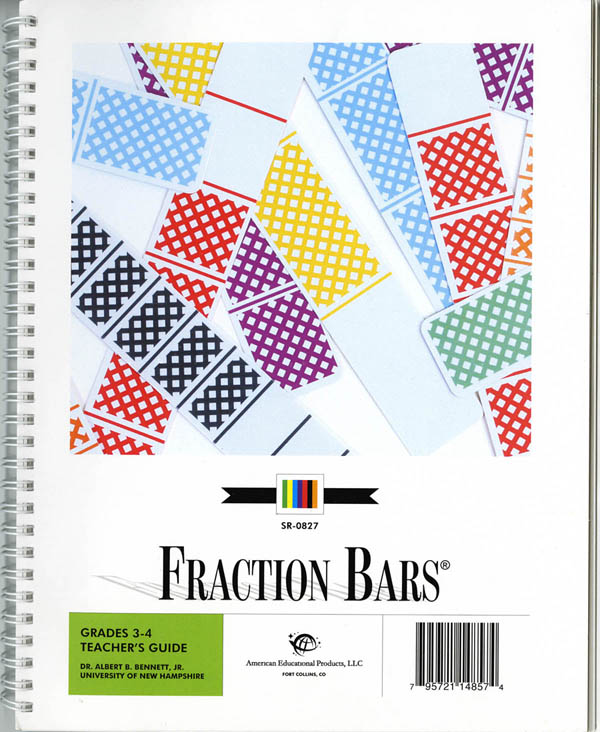(1) The Grades 3-4 Fraction Bars Teacher's Guide contains 11 lessons for grade 3 and 24 lessons for grade 4. Each step or lesson is conveniently placed on two facing pages, and all program components such as worksheets, games and materials are referenced at the point-of-use in each step. Games for use with the Fraction Bars and Fraction Playing Cards are contained within the lessons. At the end of the Guide are black and white masters to supplement some of the lessons. The lessons include the following topics: fractions for regions; equality and common denominators; word problems involving fractions for regions; inequality of fractions; fractions for collections; word problems involving fractions for collections; number lines and mixed numbers; measuring with fractions and rounding; fractions and percents; addition of fractions; subtraction of fractions; multiplication of fractions times fractions; and probability and predictions.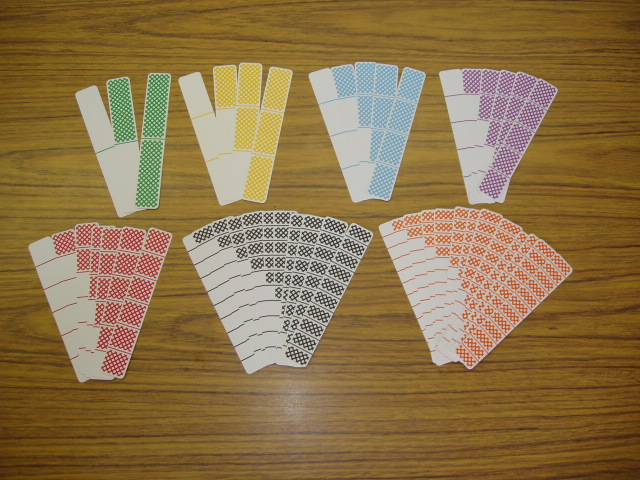(2) Each set of 49 plastic washable Fraction Bars contains bars for halves with 2 equal parts (green); bars for thirds with 3 equal parts (yellow); bars for fourths with 4 equal parts (blue); bars for fifths with 5 equal parts (purple); bars for sixths with 6 equal parts (red); bars for tenths with 10 equal parts (white); and bars for twelfths with 12 equal parts (orange). Fraction Bars are used for the activities and games in the Grades 3-4 Fraction Bars Teacher's Guide.(3) Each of the Fraction Bars Playing Cards in this color-coded 49-card deck contains a fraction and its name which corresponds to one of the Fraction Bars. The colored numeral on the playing card indicates the number of shaded parts and the total number of parts in the corresponding bar and provides for a gradual transition from the Fraction Bars model to the use of fraction symbols.(4) The Grades 3-4 Teacher Resource Package correlates directly with the lessons in the Grades 3-4 Teacher's Guide. The Grade 3 and Grade 4 sections of this package contain Activity Sheets, Performance Tests, and answers.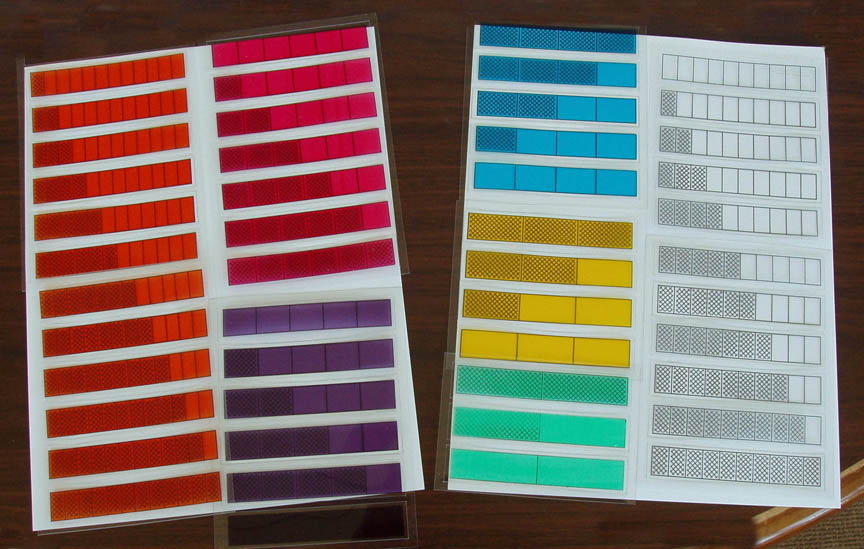(5) There are 49 colored Fraction Bars Transparencies. There is a transparency corresponding to each plastic Fraction Bar. The transparencies can be used for teacher or student demonstrations of fraction equalities, inequalities, and the four basic operations on fractions. For organizing the transparencies for classroom demonstrations and storage there is a Fraction Bars Transparency Holder.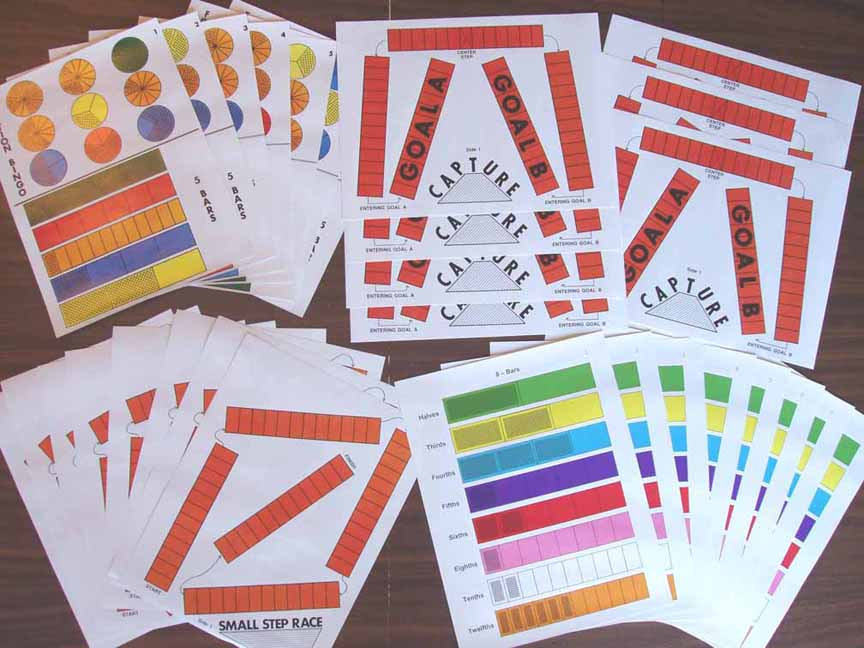(6) The 30 Fraction Bars Activity Mats are all the mats necessary for games and activities in the Grades 3-4 Fraction Bars Teacher's Guide. The complete set includes 15 Small Step/5-Bars & Fraction Bingo mats, and 15 Capture/8-Bars mats. The activities and games for these mats help to provide for a gradual nonthreatening transition from the concrete level to the use of symbols and rules for computing with fractions.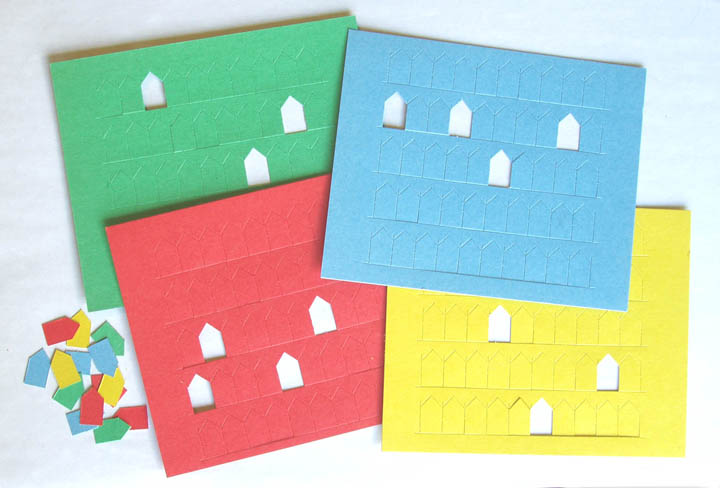(7) There are 200 game markers on punch-out sheets of cardstock in four colors.